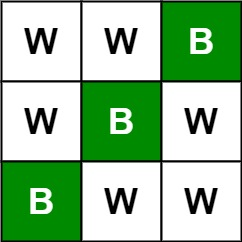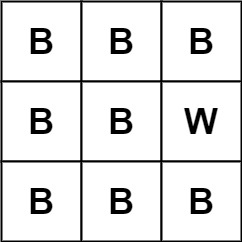### LeetCode• ㊗️
• 大家
• offer
• 多多！

## Problem

Given an m x n picture consisting of black ‘B’ and white ‘W’ pixels, return the number of black lonely pixels.

A black lonely pixel is a character ‘B’ that located at a specific position where the same row and same column don’t have any other black pixels.

Example 1:Input: picture = [["W","W","B"],["W","B","W"],["B","W","W"]]
Output: 3
Explanation: All the three 'B's are black lonely pixels.


Example 2:Input: picture = [["B","B","B"],["B","B","B"],["B","B","B"]]
Output: 0


Constraints:

• m == picture.length
• n == picture[i].length
• 1 <= m, n <= 500
• picture[i][j] is ‘W’ or ‘B’.

## Code

73. Set Matrix Zeroes

class Solution {
public int findLonelyPixel(char[][] picture) {
int m = picture.length;
int n = picture.length;

int res = 0;
// check col 0 and row 0
for (int i = 0; i < m; i++) {
if(checkLonelyPixel(picture, i, 0)) res++;
}

for (int j = 1; j < n; j++) {
if(checkLonelyPixel(picture, 0, j)) res++;
}

// B -> 1, W -> 0
for (int i = 0; i < m; i++) {
picture[i] = (picture[i] == 'B' ? '1' : '0');
}

for (int j = 0; j < n; j++) {
picture[j] = (picture[j] == 'B' ? '1' : '0');
}

for (int i = 1; i < m; i++) {
for (int j = 1; j < n; j++) {
if (picture[i][j] == 'B') {
picture[i]++;
picture[j]++;
}
}
}

for (int i = 1; i < m; i++) {
for (int j = 1; j < n; j++) {
if (picture[i][j] == 'B') {
if (picture[j] == '1' && picture[i] == '1') {
res++;
}
}
}
}

return res;
}

private boolean checkLonelyPixel(char[][] picture, int x, int y) {
if(picture[x][y] != 'B') return false;

int res = 1;

// check y column
for (int i = 0; i < picture.length; i++) {
if(i != x && picture[i][y] == 'B') res++;
}

// check x row
for (int j = 0; j < picture.length; j++) {
if(j != y && picture[x][j] == 'B') res++;
}

return res == 1;
}
}